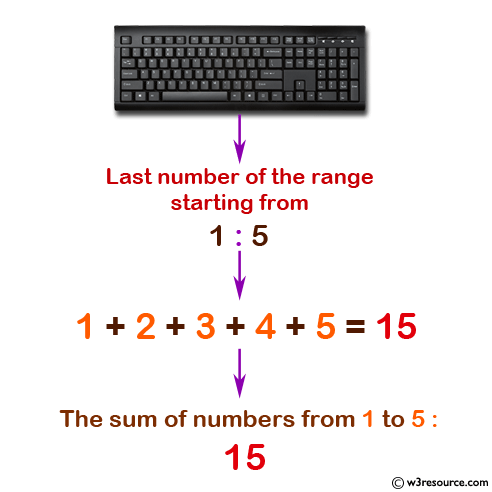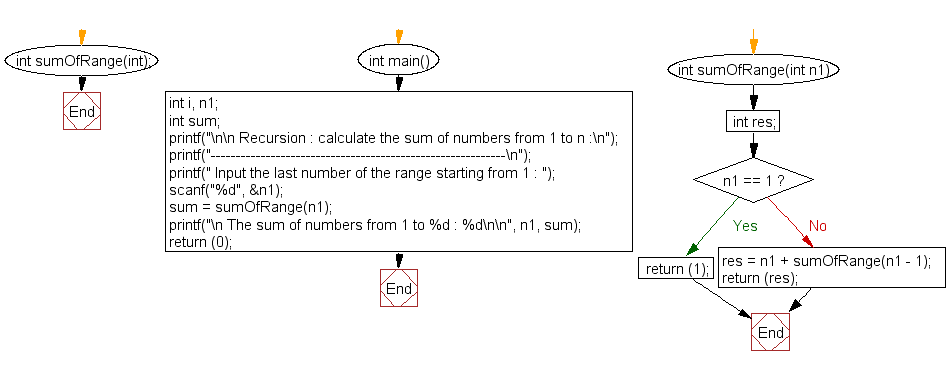﻿ C Program: Calculate the sum of numbers from 1 to n - w3resource

# C Exercises: Calculate the sum of numbers from 1 to n

## C Recursion : Exercise-2 with Solution

Write a program in C to calculate the sum of numbers from 1 to n using recursion.

Pictorial Presentation:Sample Solution:

C Code:

``````#include<stdio.h>

int sumOfRange(int);

int main()
{
int n1;
int sum;
printf("\n\n Recursion : calculate the sum of numbers from 1 to n :\n");
printf("-----------------------------------------------------------\n");

printf(" Input the last number of the range starting from 1 : ");
scanf("%d", &n1);

sum = sumOfRange(n1);
printf("\n The sum of numbers from 1 to %d : %d\n\n", n1, sum);

return (0);
}

int sumOfRange(int n1)
{
int res;
if (n1 == 1)
{
return (1);
} else
{
res = n1 + sumOfRange(n1 - 1); //calling the function sumOfRange itself
}
return (res);
}
```
```

Sample Output:

``` Recursion : calculate the sum of numbers from 1 to n :
-----------------------------------------------------------
Input the last number of the range starting from 1 : 5

The sum of numbers from 1 to 5 : 15
```

Explanation:

```int sumOfRange(int n1)
{
int res;
if (n1 == 1)
{
return (1);
} else
{
res = n1 + sumOfRange(n1 - 1); //calling the function sumOfRange itself
}
return (res);
}
```

The above function sumOfRange() calculates the sum of all natural numbers from 1 to the given number n1. It uses a recursive approach to calculate the sum, where if the number n1 is 1, the function returns 1, otherwise it adds n1 to the sum of all natural numbers from 1 to n1-1 and returns the result.

Time complexity and space complexity:

The time complexity of this function is O(n), where n is the given input number n1. This is because the function makes n recursive calls, each with a constant time complexity of O(1).

The space complexity of this function is also O(n), because the function creates n activation records on the call stack, one for each recursive call.

Flowchart:C Programming Code Editor:

Have another way to solve this solution? Contribute your code (and comments) through Disqus.

What is the difficulty level of this exercise?

Test your Programming skills with w3resource's quiz.

﻿

## C Programming: Tips of the Day

__FILE__ macro shows full path

```#include <string.h>

#define __FILENAME__ (strrchr(__FILE__, '/') ? strrchr(__FILE__, '/') + 1 : __FILE__)
For Windows use '\\' instead of '/'.
```

Ref : https://bit.ly/3iEWRoT

We are closing our Disqus commenting system for some maintenanace issues. You may write to us at reach[at]yahoo[dot]com or visit us at Facebook